# Eight palm

There grows 8 palms by the sea. At the first sitting one parrot, on second two, on third sits four parrots on each other twice the previous parrots sitting on a previous palm. How many parrots sitting on eighth palm?

Result

n =  128

#### Solution:Leave us a comment of example and its solution (i.e. if it is still somewhat unclear...):

Showing 0 comments:Be the first to comment!## Next similar examples:

1. GP - 8 itemsDetermine the first eight members of a geometric progression if a9=512, q=2
2. Geometric progression 2There is geometric sequence with a1=5.7 and quotient q=-2.5. Calculate a17.
3. Gp - 80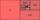Sum of the first four members of a geometric progression is 80. Determine they if we know that the fourth member is nine times greater than the second.
4. Geometric sequence 4It is given geometric sequence a3 = 7 and a12 = 3. Calculate s23 (= sum of the first 23 members of the sequence).
5. Five membersWrite first 5 members geometric sequence and determine whether it is increasing or decreasing: a1 = 3 q = -2
6. Six termsFind the first six terms of the sequence a1 = -3, an = 2 * an-1
7. Quotient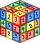Determine the quotient and the second member of the geometric progression where a3=10, a1+a2=-1,6 a1-a2=2,4.
8. Piano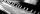If Suzan practicing 10 minutes at Monday; every other day she wants to practice 2 times as much as the previous day, how many hours and minutes will have to practice on Friday?
9. Theorem proveWe want to prove the sentense: If the natural number n is divisible by six, then n is divisible by three. From what assumption we started?
10. Tenth memberCalculate the tenth member of geometric sequence when given: a1=1/2 and q=2
11. One halfOne half of ? is: ?
12. PowersExpress the expression ? as the n-th power of the base 10.
13. Ray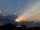Light ray loses 1/19 of brightness passing through glass plate. What is the brightness of the ray after passing through 7 identical plates?
14. Compare IIWhich of the numbers 710, 107 is greater?
15. Linear systemSolve a set of two equations of two unknowns: 1.5x+1.2y=0.6 0.8x-0.2y=2
16. Geometric progression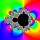Fill 4 numbers between 4 and -12500 to form geometric progression.
17. Price increase 2xIf two consecutive times we increase the price of the product by 20%, how many % is higher final price than the original?